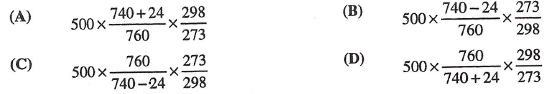# Problem: A 500 mL gas sample is collected over water at a pressure of 740 mmHg and 25°C. What is the volume of the dry gas at STP? (STP= 1 atm and 0°C.)Vapor Pressure of H2O at 25°C: 24 mmHg

###### FREE Expert Solution
87% (390 ratings)
###### FREE Expert Solution

We’re being asked to determine the volume of the gas sample collected over water at STP (standard temperature and pressure).

At STP:         T = 0°C

P = 1 atm

We’re going to calculate the volume of the gas using the ideal gas equation.

$\overline{){\mathbf{P}}{\mathbf{V}}{\mathbf{=}}{\mathbf{n}}{\mathbf{R}}{\mathbf{T}}}$

P = pressure, atm
V = volume, L
n = moles, mol
R = gas constant = 0.08206 (L·atm)/(mol·K)
T = temperature, K

The amount of gas (n) will not change and R is a constant:

$\mathbit{P}\mathbit{V}\mathbf{=}\overline{)\mathbf{n}\mathbf{R}}\mathbit{T}\phantom{\rule{0ex}{0ex}}\frac{\mathbf{P}\mathbf{V}}{\mathbf{T}}\mathbf{=}\frac{\overline{)\mathbf{T}}}{\overline{)\mathbf{T}}}\phantom{\rule{0ex}{0ex}}\frac{\mathbf{P}\mathbf{V}}{\mathbf{T}}\mathbf{=}\mathbf{1}$

Relating the two conditions:

87% (390 ratings)###### Problem Details

A 500 mL gas sample is collected over water at a pressure of 740 mmHg and 25°C. What is the volume of the dry gas at STP? (STP= 1 atm and 0°C.)

Vapor Pressure of H2O at 25°C: 24 mmHgWhat scientific concept do you need to know in order to solve this problem?

Our tutors have indicated that to solve this problem you will need to apply the Collecting Gas Over Water concept. If you need more Collecting Gas Over Water practice, you can also practice Collecting Gas Over Water practice problems.

What is the difficulty of this problem?

Our tutors rated the difficulty ofA 500 mL gas sample is collected over water at a pressure of...as high difficulty.

How long does this problem take to solve?

Our expert Chemistry tutor, Jules took 4 minutes and 13 seconds to solve this problem. You can follow their steps in the video explanation above.

What professor is this problem relevant for?

Based on our data, we think this problem is relevant for Professor Velazquez's class at UCD.